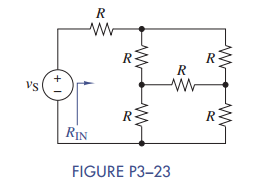Chat Now

# Use simple engineering intuition to find the input resistance of the circuit in Figure P3−23....

### Use simple engineering intuition to find the input resistance of the circuit in Figure P3−23....

Use simple engineering intuition to find the input resistance of the circuit in Figure P3−23. Use either node-voltage or mesh-current analysis to prove your intuition.manish jayant 28-Jul-2020Get solution